# Chemistry Assignment Help With Mixing Of Two Volatile Liquids

1. Home
2. Chemistry
3. Liquid Solution
4. apour Pressure
5. Volatile Liquids

## Mixing Of Two Volatile Liquids

Let two volatile liquids A and B dissolve each other to form an ideal solution. Then the vapours above the solution will contain the vapours of A and B.

Applying Dalton’s law of partial pressure the total vapour pressure of the solution will be

Psolution = PA + PB

Where PA and PB are the partial vapour pressures of A and B.

Solution being dilute (Condition for ideality) Raoult's law can be applied.

Raoult’s law for binary solutions state that “the vapour pressure of any volatile constituent of a binary solution at any given temperature is equal to the product of the vapour pressure of pure constituent and its mole fraction”.PA = PºA XA

PB = PºB XB

PºA and PºB are vapour pressures of pure A and B respectively.

cA and cB are mole fraction of A and B in liquid solution respectively

Hence

Ps =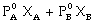Hence,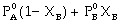Ps =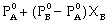This equation is of the form y = mx + c

The value of m (slope) may be (+ve) or (–ve) depending upon whether PB0 > PA0 (m = + ve) or

PB0 < PA0 (m = –ve).

Hence a plot of a graph of Ps versus XB will be a straight line with an intercept on y-axis equal to pA and slope equal to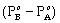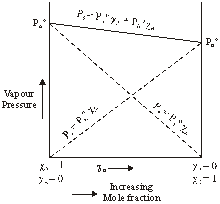### Vapour composition

Vapour composition means to find out the mole fraction of A and B in vapour. Dalton’s law of particle pressure is used to calculate the vapour composition, as we know

Partial pressure = mole fraction × total pressure

PA =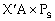or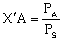Similarly for B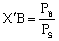where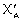and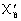are mole fraction of ‘A’ and ‘B’ in vapour phase and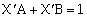### Homework Help For Mixing of two volatile liquids

assignmenthelp.net provides best Online Assignment Help service in chemistry for all standards. Our Tutor provide their high quality and optimized Tutorial help to fulfill all kind of need of Students.